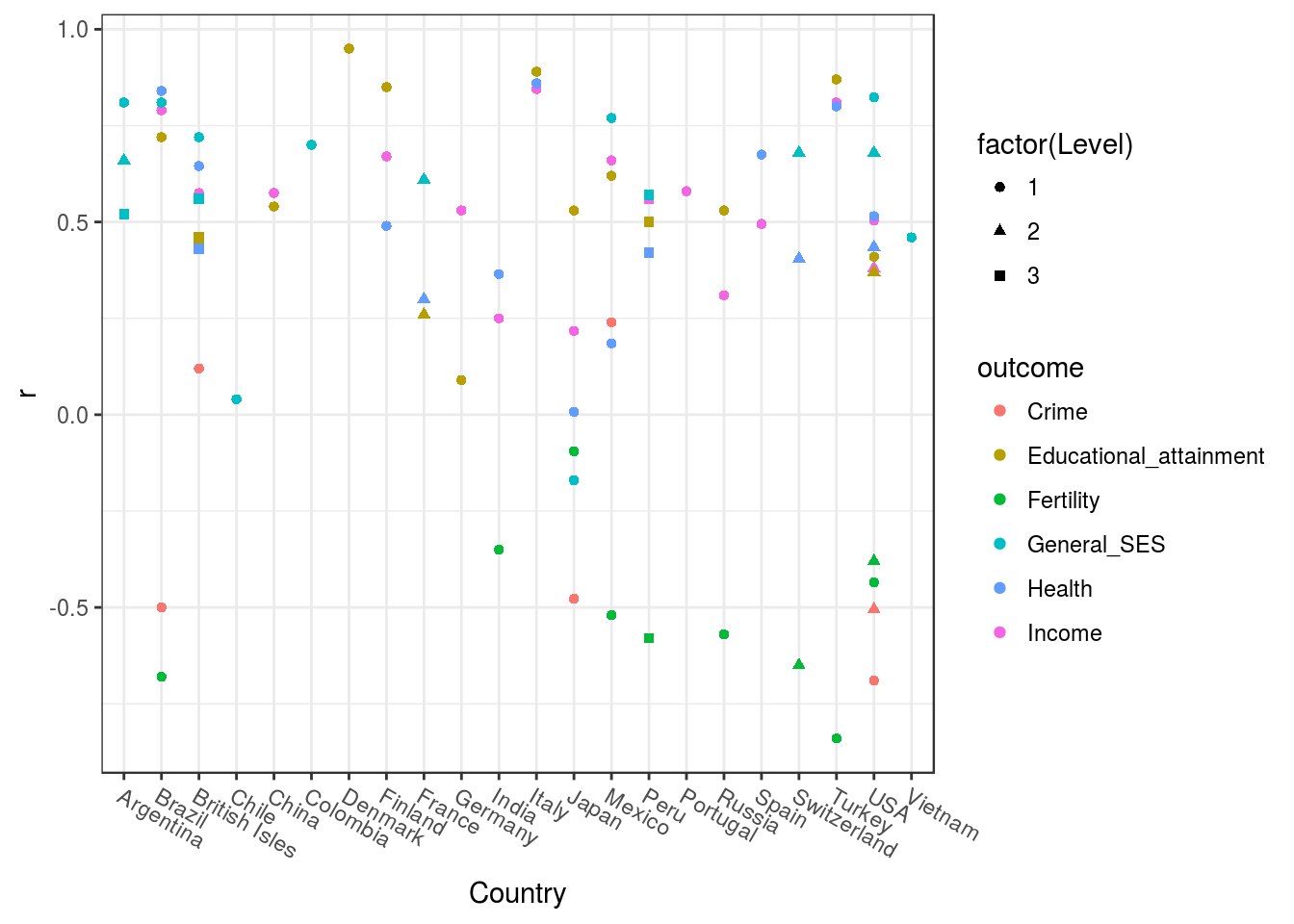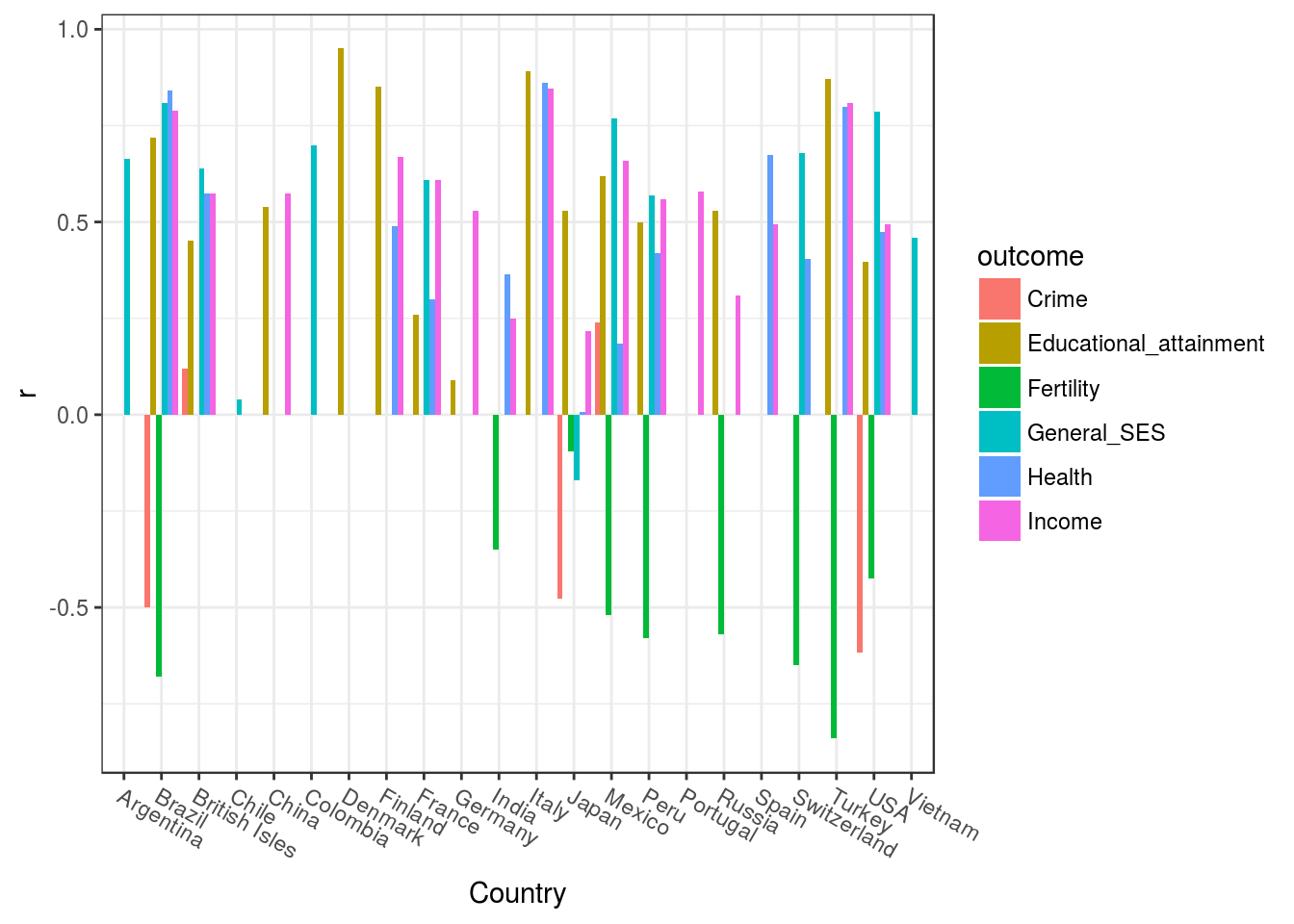Init

options(digits = 2)
library(pacman)
p_load(kirkegaard, googlesheets, plyr, metafor)

Data

#auth
gs_auth()

gs = gs_url("https://docs.google.com/spreadsheets/d/1drrchXhJs5elWCvtYCOwMX7zG22_A_WD73KcAyBfTn4/edit#gid=211583593")
## Sheet-identifying info appears to be a browser URL.
## googlesheets will attempt to extract sheet key from the URL.
## Putative key: 1drrchXhJs5elWCvtYCOwMX7zG22_A_WD73KcAyBfTn4
## Sheet successfully identified: "Lynn et al, Regional IQ studies review, 2017, Intelligence, dataset"
d = gs_read(gs) %>% df_legalize_names()
## Accessing worksheet titled 'data'.
## Warning: Missing column names filled in: 'X20' , 'X21' 
## Parsed with column specification:
## cols(
##   .default = col_double(),
##   Country = col_character(),
##   Name = col_character(),
##   Level = col_integer(),
##   Unit N = col_integer(),
##   Population 2010 = col_number(),
##   Authors = col_character(),
##   X20 = col_character(),
##   X21 = col_character(),
##   Study Number = col_integer()
## )
## See spec(...) for full column specifications.

Functions

#ad hoc functions
rowMeans(cbind(x, y), na.rm = T) %>% plyr::mapvalues(NaN, NA)
}

#vec2df
vec2df = function(x) {
y = x %>% as.data.frame() %>% t() %>% as.data.frame()
names(y) = names(x)
y
}

#NaN2NA
NaN2NA = function(x) {
x[is.nan(x)] = NA
x
}

#remove NAs from output, hack solution
removeNAs = function(x) {
#convert all to strings
x %>% map_df(~as.character(.) %>% mapvalues(NA, ""))
}

Basic stats

#unique studies
length(unique(d$Authors)) ##  45 #overview of levels table2(d$Level)
#count by country
table2(d$Country) Simplify and aggregate To avoid double counting and redundant data. #first, average the alternate columns d2 = d %>% mutate( Income = add_vectorized(Per_capita_income_1, Per_capita_income_2), Educational_attainment = add_vectorized(Educational_attainment_1, Educational_attainment_2), Life_expectancy = add_vectorized(Life_expectancy_1, Life_expectancy_2), Infant_mortality = add_vectorized(Infant_mortality_1, Infant_mortality_2), Fertility = add_vectorized(Fertility_1, Fertility_2), Crime = add_vectorized(Crime_1, Crime_2), Health = add_vectorized(Life_expectancy, Infant_mortality*-1) ) #vars primary_vars = c("Income", "Educational_attainment", "Fertility", "Crime", "Health", "General_SES") #aggregate without countries d2_country = ddply(d2, "Country", function(x) { #begin with a copy y = x[1, c("Country", "Population_2010")] #add n studies y$Study_N = nrow(x)

#add average data for numeric cols of interest
y2 = colMeans(x[c("Unit_N", primary_vars)], na.rm = T)  %>% NaN2NA() %>% vec2df()

cbind(y, y2)
})

#aggregate without countries
d2_country_levels = ddply(d2, c("Country", "Level"), function(x) {
#begin with a copy
y = x[1, c("Country", "Level", "Population_2010")]

y$Study_N = nrow(x) #add average data for numeric cols of interest y2 = colMeans(x[c("Unit_N", primary_vars)], na.rm = T) %>% NaN2NA() %>% vec2df() cbind(y, y2) }) #output aggregated tables back to spreadsheet gs_edit_cells(gs, ws = "aggregated_country", input = d2_country %>% removeNAs()) ## The following from values were not present in x: NA ## The following from values were not present in x: NA ## The following from values were not present in x: NA ## The following from values were not present in x: NA ## Range affected by the update: "R1C1:R23C10" ## Worksheet "aggregated_country" successfully updated with 230 new value(s). gs_edit_cells(gs, ws = "aggregated_country_level", input = d2_country_levels %>% removeNAs()) ## The following from values were not present in x: NA ## The following from values were not present in x: NA ## The following from values were not present in x: NA ## The following from values were not present in x: NA ## The following from values were not present in x: NA ## Range affected by the update: "R1C1:R27C11" ## Worksheet "aggregated_country_level" successfully updated with 297 new value(s). Meta-analyze #simple means colMeans(d2_country[primary_vars], na.rm = T) ## Income Educational_attainment Fertility ## 0.56 0.59 -0.52 ## Crime Health General_SES ## -0.25 0.49 0.55 colMeans(d2_country_levels[primary_vars], na.rm = T) ## Income Educational_attainment Fertility ## 0.55 0.56 -0.51 ## Crime Health General_SES ## -0.30 0.49 0.58 #weighted by population map_df(d2_country[primary_vars], ~wtd_mean(., w = d2_country$Population_2010))
#weighted by sqrt population
map_df(d2_country[primary_vars], ~wtd_mean(., w = d2_country$Population_2010 %>% sqrt())) #weighted by sqrt N divisions map_df(d2_country[primary_vars], ~wtd_mean(., w = d2_country$Unit_N %>% sqrt()))

Figures

#overall
d2_country_levels %>%
gather_("outcome", "r", primary_vars) %>%
ggplot(aes(Country, r, color = outcome, shape = factor(Level), group = outcome)) +
geom_point(size = 1.5) +
theme_bw() +
theme(axis.text.x = element_text(angle = -30, hjust = 0))
## Warning: Removed 76 rows containing missing values (geom_point).GG_save("figs/overview.png")
## Warning: Removed 76 rows containing missing values (geom_point).
#bars
d2_country %>%
gather_("outcome", "r", primary_vars) %>%
ggplot(aes(Country, r, fill = outcome)) +
geom_bar(stat = "identity", position = "dodge") +
theme_bw() +
theme(axis.text.x = element_text(angle = -30, hjust = 0))
## Warning: Removed 63 rows containing missing values (geom_bar).GG_save("figs/overview2.png")
## Warning: Removed 63 rows containing missing values (geom_bar).